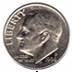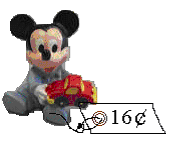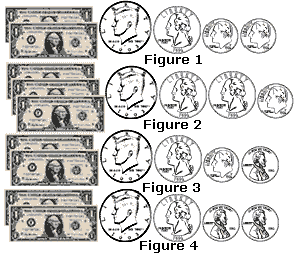Related Links

## Definition Of Value

The numerical worth of an object is known as its value.

### Examples of Value

The coin shown is a dime and its value is 10 cents.The value of the Mickey mouse shown in tag is 16 cents.### Solved Example on Value

#### Ques: Tom needs $2.95 to buy a gift for his friend. Which combination of bills and coins shows the amount$2.95?##### Choices:

A. Figure 3
B. Figure 4
C. Figure 1
D. Figure 2
Correct Answer: C

#### Solution:

Step 1: The value of the bills and coins in
Figure 1 is $2 + 50 cents + 25 cents + 10 cents + 10 cents =$2.95. [1 dollar = 100 cents, 1 quarter = 25 cents, 1 dime = 10 cents.]
Step 2: The value of the bills and coins in
Figure 2 is $3 + 50 cents + 25 cents + 25 cents + 10 cents =$4.10. [1 dollar = 100 cents, 1 quarter = 25 cents, 1 dime = 10 cents.]
Step 3: The value of the bills and coins in
Figure 3 is $2 + 50 cents + 25 cents + 10 cents + 1 cent =$2.86. [1 dollar = 100 cents, 1 quarter = 25 cents, 1 dime = 10 cents, 1 penny = 1 cent.]
Step 4: The value of the bills and coins in
Figure 4 is $2 + 50 cents + 25 cents + 1 cent + 1 cent =$2.77. [1 dollar = 100 cents, 1 quarter = 25 cents, 1 dime = 10 cents, 1 penny = 1 cent.]
Step 5: So, the combination of bills and coins in Figure 1 count up to \$2.95.